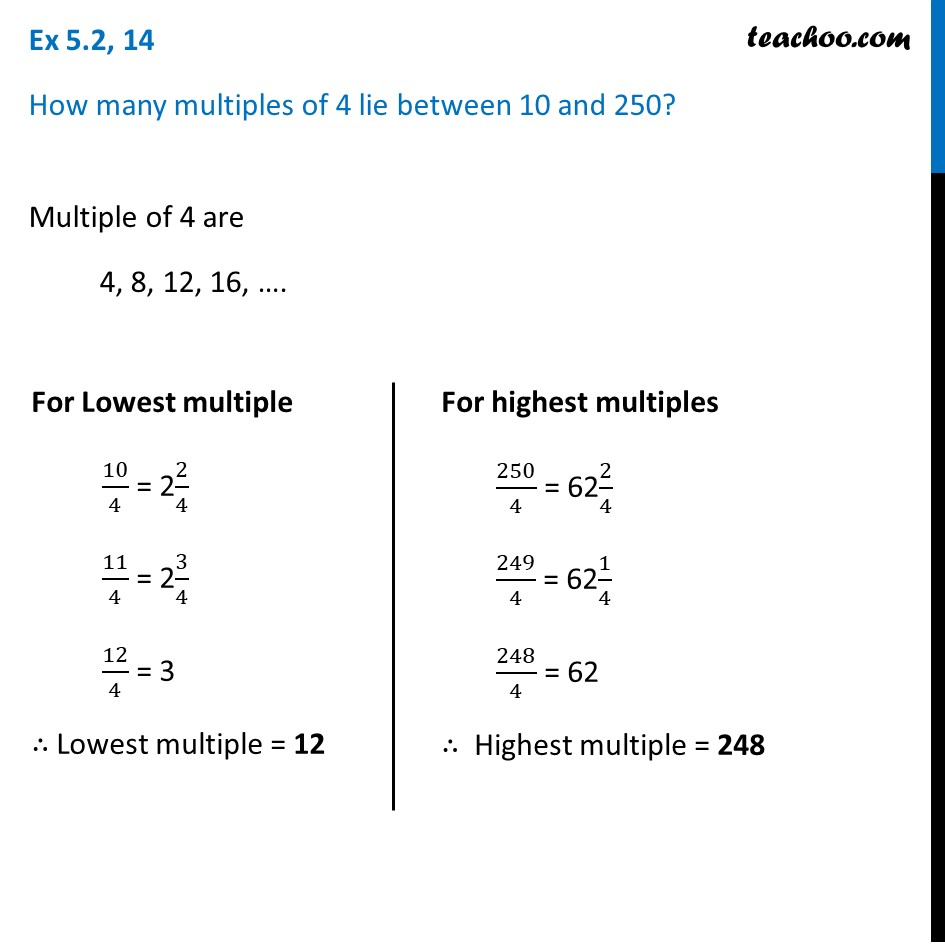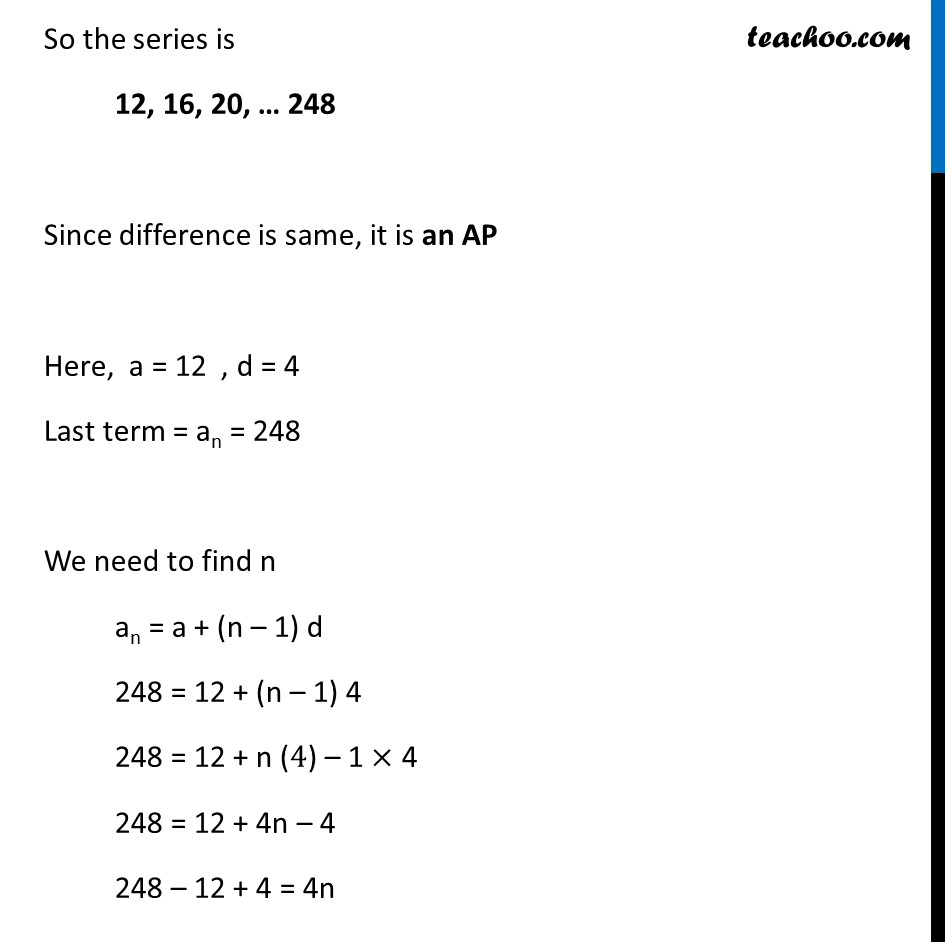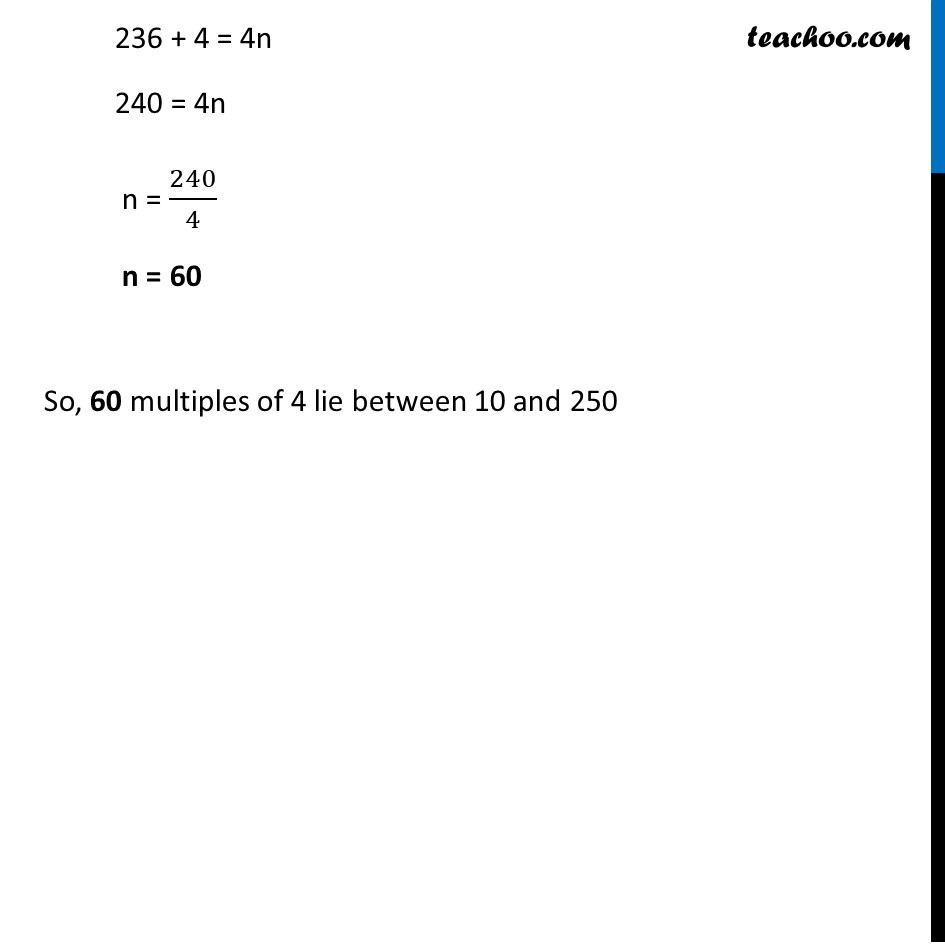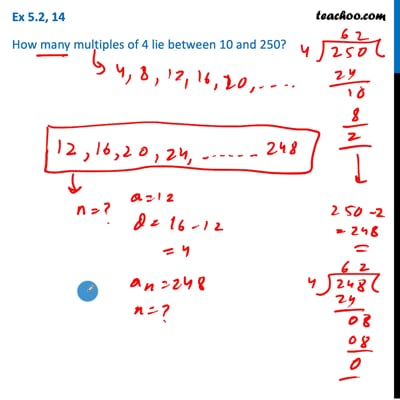Ex 5.2

Chapter 5 Class 10 Arithmetic Progressions
Serial order wiseThis video is only available for Teachoo black users

Maths Crash Course - Live lectures + all videos + Real time Doubt solving!

### Transcript

Ex 5.2, 14 How many multiples of 4 lie between 10 and 250? Multiple of 4 are 4, 8, 12, 16, …. For Lowest multiple 10/4 = 22/4 11/4 = 23/4 12/4 = 3 ∴ Lowest multiple = 12 For highest multiples 250/4 = 622/4 249/4 = 621/4 248/4 = 62 ∴ Highest multiple = 248 So the series is 12, 16, 20, … 248 Since difference is same, it is an AP Here, a = 12 , d = 4 Last term = an = 248 We need to find n an = a + (n – 1) d 248 = 12 + (n – 1) 4 248 = 12 + n (4) – 1 × 4 248 = 12 + 4n – 4 248 – 12 + 4 = 4n 236 + 4 = 4n 240 = 4n n = 240/4 n = 60 So, 60 multiples of 4 lie between 10 and 250